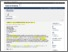Introduction to nonlinear discrete systems: theory and modelling

Tsoy, Eduard N. and Umarov, Bakhram A. (2018) Introduction to nonlinear discrete systems: theory and modelling. European Journal of Physics, 39 (5). pp. 1-13. ISSN 0143-0807 E-ISSN 1361-6404PDF - Published Version
Restricted to Repository staff onlyPDF (Scopus) - Supplemental Material
Restricted to Repository staff onlyPreview
PDF

Abstract

An analysis of discrete systems is important for understanding of various physical processes, such as excitations in crystal lattices and molecular chains, the light propagation in waveguide arrays, and the dynamics of Bose-condensate droplets. In basic physical courses, usually the linear properties of discrete systems are studied. In this paper we propose a pedagogical introduction to the theory of nonlinear distributed systems. The main ideas and methods are illustrated using a universal model for different physical applications, the discrete nonlinear Schrödinger (DNLS) equation. We consider solutions of the DNLS equation and analyse their linear stability. The notions of nonlinear plane waves, modulational instability, discrete solitons and the anti-continuum limit are introduced and thoroughly discussed. A Mathematica program is provided for better comprehension of results and further exploration. Also, a few problems, extending the topic of the paper, for independent solution are given.

Item Type: Article (Journal) 3938/67671 Arrays; Chains; Discrete nonlinear schrodinger equation; Discrete solitons; Discrete systems; lattices; Mathematica code Q Science > QA Mathematics > QA297 Numerical AnalysisQ Science > QC Physics Kulliyyah of Science > Department of Physics Dr Bakhram Umarov 27 Nov 2018 16:25 10 Apr 2019 15:45 http://irep.iium.edu.my/id/eprint/67671View Item